# Functions in C++

## Functions in C++ with Examples:

In this article, I am going to give you a brief introduction to Functions in C++ Language with examples. Please read our previous section, where we discussed Strings in C++ with examples. Functions are one of the powerful features of any programming language.

##### What is a Function in C++?

A function is a piece of program code that performs a specific task. It can be a small task or a big task but the function will perform that task completely. Functions take some input as parameters and return the result as a return value.

Functions are useful for procedural programming or modular programming. If we write a function then we can reuse it in the program. Multiple times we can use a function inside a program. Even we can use it in other programs.

The collection of functions is known as a library. The C/C++ languages provide a lot of built-in functions in their library and we commonly used them for making the programming easy. Let us see how we can write functions and see the idea behind writing the functions.

void main(){
}

Every C++ program must have a main function. Here we have written void main even we can write as int main(). And we also know that this is the entry point of our program and our program starts execution from here only. And whatever we want, we can write inside the curly braces of the main function.

Suppose if we have a very big program let’s say we have some 10000 lines of code. Then everything we are writing inside the main function only and if we have a single function then this style of programming is called monolithic programming. If we write the program like this, let us see the problems we will face.

##### Problems in Monolithic Programming:
• First problem: if there is an error in a single line then it’s an error in the entire program or entire main function.
• Second problem: 10000 lines of code, we cannot finish it in one hour or one day, it might take few days and throughout the time we should remember everything. Then only we can make changes or write new lines in the program. So, we should memorize the whole program.
• Third problem: How many people can write this one single main function with? Only one person can write. We cannot make it as teamwork and more than one people can’t work on the same main function. So, work cannot be distributed in a team.
• Fourth problem: when this program becomes very big, it may fit in some computer memories and it may not fit in some of the memories. It Depends on the size and depends on the hardware contribution of the computer on which you are running.

So, these are the few problems due to monolithic programming. Monolithic means everything is a single unit.

We prefer to break the program into pieces, manageable and small pieces, and re-usable pieces. The benefit of this is we can develop piece-wise so we can concentrate on one piece of code at a time. The second thing is pieces can be distributed among the team of programmers and they can develop some set of pieces and we can collect them together and make it as a single program.

And the main will be integrating all of them directly or indirectly. One more thing is when we are on some computer then we can load and unload the pieces that we require. So, we don’t have to have a very large-sized memory on the computer in which we are running, so all the problems are solved. This is nothing but modular programming. This can be done using functions.

##### How to write a function in C++?

So let us see how to write a function. First of all, the function should have a name. Then it should have a parameter list or also called an argument list (the parameters it is taking), then the function should have a return type. For better understanding, please have a look at the below image.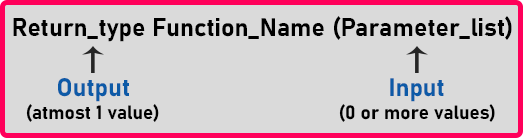• Functions are identified by their name. A function can take 0 or more parameters means it may not take any input.
• A function may or may not return a value but it can return at most one value. It cannot return multiple values but it can take multiple values as parameters. If the function is not returning any value, then the return type should be void.
• The rules for giving function names are the same as the rules for giving the variable names. Same rules you should follow for giving function names also.

Now let us write one function and use it in our main function.

void display(){
cout << “Hello”;
}
void main(){
display();
}

Here we have a function called display() which will print Hello when it is called. This function is taking no arguments. We have called this function inside the main() function. So let us see how it runs.

When the main function starts, it will come to the line where we have called the display function, then the control will go to the display function, and then it will execute the cout line. After executing the print line, the control will again return back and continue the main function.

Now one important thing that inside the functions avoid user interaction that is ‘cin’ and ‘cout’. It’s a bad function. So the idea behind that is to suppose there is an organization and ‘main’ function is a manager or the owner of a business and ‘display()’ is his employee.

So, who called the employee? The manager has called the employee for some work. So, who should contact or who should communicate with the clients of a business or the customers of a business? The manager or the owner should communicate with the client. So ‘cin’ and ‘cout’ are like communication with the client.

So, communication client is whose client? User. So, through the main function, only the communication with the user should be done. All other functions must just support the main function. They should not have ‘cin’ and ‘cout’ in them. This is a good practice. We should avoid it.

##### Function for Adding Two Numbers in C++.

We are writing the function for adding two numbers.

int add (int x, int y){
int z;
z = a + b;
return z;
}

This function is taking two parameters x and y of integer type. Inside this function, we have taken an extra variable that is z. And store the result of a + b in z variable and return this z. Now let us write main function.

void main (){
int a = 3, b = 4, c;
c = add (a + b);
cout << c << endl;
}

Inside the main function, we have taken 3 variables. Next, we have called the function ‘add’ with ‘a’ and ‘b’ as parameters and store the result in ‘c’. And print the value of ‘c’. Now let us look at how the memory is created for the above functions.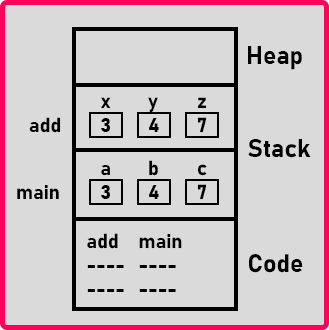This is the memory structure of our program. As you can see two activation records are there. One for the variables which we have declared in the ‘main’ function and another for the variables which we declared inside the ‘add’ function.

So, when we call the ‘add’ function, an activation record will be created inside the memory and when the add function completes its execution then the activation record will be deleted from the stack memory. That means once the function completes its execution, then all the memory which is consumed by that function in the stack area will be cleared automatically.

Memory in the heap will not be deallocated automatically. The function should release it by saying delete, so if memory is in heap, it must be deleted. Now let us look at the complete program.

##### Program1: Sample Program to show the use of Functions in C++
```#include <iostream>
using namespace std;
void Display()
{
cout << "Hello";
}

int main()
{
Display ();
return 0;
}
```
###### Output: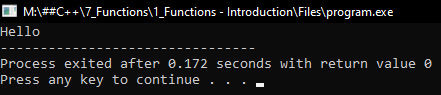##### Program 2: Program to add two numbers using Functions in C++
```#include <iostream>
using namespace std;
{
float z;
z = x + y;
return z;
}

int main()
{
float x = 23.3, y = 6.5, z;
cout << z << endl;
return 0;
}
```
###### Output: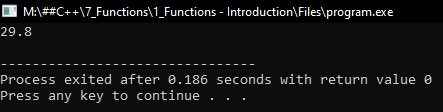##### Program 2: Program to find the maximum of three numbers using Functions in C++
```#include <iostream>
using namespace std;
int Maximum(int a, int b, int c)
{
if (a > b && a > c)
return a;
else if (b > c)
return b;
else
return c;
}

int main()
{
int x = 10, y = 15, z = 20, r;
r = Maximum(x, y, z);
cout << r << endl;
return 0;
}
```
###### Output: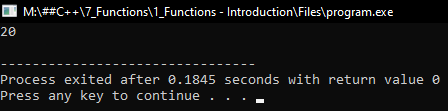##### Will the functions occupy space in memory?

Yes, the machine code of a function is kept code section.

##### Will a function occupy space even if it is not called?

Yes, if a function is defined in a program or included from the library, it will occupy space in the code section.

##### Where the memory for the variable of a function is created?

Memory for the variables used in a function is created in the stack.

##### When the memory for variables will be allocated?

Memory for the variables will be allocated at runtime when the function is called and deleted when the function ends.

##### Is the memory for variables is allocated freshly for each call?

For “for each” call of a function memory for the variables is created freshly in the stack.

##### What is the return type of a function?

When a function is called by passing parameters, it will compute and get the results. A function can return the result to a calling function. The return type is the data type of a value return by the function.

##### What is void?

If a function is not returning any value then its return type is mentioned as void.

##### Difference between int main() and void main()
• void main() means the main function is not returning any value.
• int main() means main function will return 0; 0 is a success code.
• The function has terminated successfully. main() will return the
• value to the operating system, like windows.
• In C++ int main() is standard.